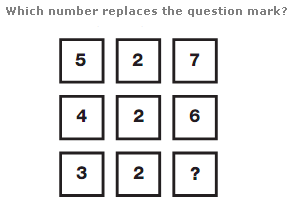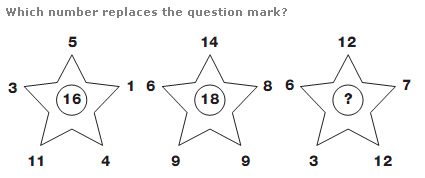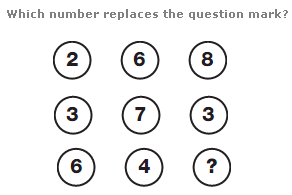# Puzzles - Number puzzles

### Exercise :: Number puzzlesAnswer : 5 Explanation : In each column of the diagram, the central value equals the average of the upper and lower numbers.Answer : 14 Explanation : In each star, the central value equals the sum of the top and lower two numbers, minus the left and right hand numbers.Answer : 1 Explanation : Read each row of 3 numbers as a 3 digit number, and add the top row value to the middle row, to give the result written in the bottom row.